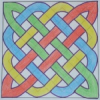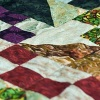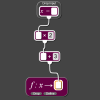# Resources tagged with: Factors and multiples

Filter by: Content type:
Age range:
Challenge level:

### There are 106 results

Broad Topics > Properties of Numbers > Factors and multiples### Take Three from Five

##### Age 11 to 16Challenge Level

Caroline and James pick sets of five numbers. Charlie chooses three of them that add together to make a multiple of three. Can they stop him?### Repeaters

##### Age 11 to 14Challenge Level

Choose any 3 digits and make a 6 digit number by repeating the 3 digits in the same order (e.g. 594594). Explain why whatever digits you choose the number will always be divisible by 7, 11 and 13.### Why 24?

##### Age 14 to 16Challenge Level

Take any prime number greater than 3 , square it and subtract one. Working on the building blocks will help you to explain what is special about your results.### Even So

##### Age 11 to 14Challenge Level

Find some triples of whole numbers a, b and c such that a^2 + b^2 + c^2 is a multiple of 4. Is it necessarily the case that a, b and c must all be even? If so, can you explain why?### Special Sums and Products

##### Age 11 to 14Challenge Level

Find some examples of pairs of numbers such that their sum is a factor of their product. eg. 4 + 12 = 16 and 4 × 12 = 48 and 16 is a factor of 48.### Summing Consecutive Numbers

##### Age 11 to 14Challenge Level

15 = 7 + 8 and 10 = 1 + 2 + 3 + 4. Can you say which numbers can be expressed as the sum of two or more consecutive integers?### Hot Pursuit

##### Age 11 to 14Challenge Level

I added together the first 'n' positive integers and found that my answer was a 3 digit number in which all the digits were the same...### Three Times Seven

##### Age 11 to 14Challenge Level

A three digit number abc is always divisible by 7 when 2a+3b+c is divisible by 7. Why?### Drawing Celtic Knots

##### Age 11 to 14Challenge Level

Here is a chance to create some Celtic knots and explore the mathematics behind them.##### Age 11 to 14Challenge Level

List any 3 numbers. It is always possible to find a subset of adjacent numbers that add up to a multiple of 3. Can you explain why and prove it?### Counting Factors

##### Age 11 to 14Challenge Level

Is there an efficient way to work out how many factors a large number has?### Beelines

##### Age 14 to 16Challenge Level

Is there a relationship between the coordinates of the endpoints of a line and the number of grid squares it crosses?### What Numbers Can We Make Now?

##### Age 11 to 14Challenge Level

Imagine we have four bags containing numbers from a sequence. What numbers can we make now?### Number Rules - OK

##### Age 14 to 16Challenge Level

Can you produce convincing arguments that a selection of statements about numbers are true?### DOTS Division

##### Age 14 to 16Challenge Level

Take any pair of two digit numbers x=ab and y=cd where, without loss of generality, ab > cd . Form two 4 digit numbers r=abcd and s=cdab and calculate: {r^2 - s^2} /{x^2 - y^2}.### Common Divisor

##### Age 14 to 16Challenge Level

Find the largest integer which divides every member of the following sequence: 1^5-1, 2^5-2, 3^5-3, ... n^5-n.### Multiplication Magic

##### Age 14 to 16Challenge Level

Given any 3 digit number you can use the given digits and name another number which is divisible by 37 (e.g. given 628 you say 628371 is divisible by 37 because you know that 6+3 = 2+7 = 8+1 = 9). . . .### Can You Find a Perfect Number?

##### Age 7 to 14### Hypotenuse Lattice Points

##### Age 14 to 16Challenge Level

The triangle OMN has vertices on the axes with whole number co-ordinates. How many points with whole number coordinates are there on the hypotenuse MN?### Big Powers

##### Age 11 to 16Challenge Level

Three people chose this as a favourite problem. It is the sort of problem that needs thinking time - but once the connection is made it gives access to many similar ideas.### Mod 3

##### Age 14 to 16Challenge Level

Prove that if a^2+b^2 is a multiple of 3 then both a and b are multiples of 3.### Inclusion Exclusion

##### Age 11 to 14Challenge Level

How many integers between 1 and 1200 are NOT multiples of any of the numbers 2, 3 or 5?### Just Repeat

##### Age 11 to 14Challenge Level

Think of any three-digit number. Repeat the digits. The 6-digit number that you end up with is divisible by 91. Is this a coincidence?##### Age 11 to 14Challenge Level

Great Granddad is very proud of his telegram from the Queen congratulating him on his hundredth birthday and he has friends who are even older than he is... When was he born?### Got It

##### Age 7 to 14Challenge Level

A game for two people, or play online. Given a target number, say 23, and a range of numbers to choose from, say 1-4, players take it in turns to add to the running total to hit their target.### What a Joke

##### Age 14 to 16Challenge Level

Each letter represents a different positive digit AHHAAH / JOKE = HA What are the values of each of the letters?##### Age 11 to 14Challenge Level

Make a set of numbers that use all the digits from 1 to 9, once and once only. Add them up. The result is divisible by 9. Add each of the digits in the new number. What is their sum? Now try some. . . .### Alison's Quilt

##### Age 11 to 14Challenge Level

Nine squares are fitted together to form a rectangle. Can you find its dimensions?### Shifting Times Tables

##### Age 11 to 14Challenge Level

Can you find a way to identify times tables after they have been shifted up or down?### Counting Cogs

##### Age 7 to 14Challenge Level

Which pairs of cogs let the coloured tooth touch every tooth on the other cog? Which pairs do not let this happen? Why?### Exploring Simple Mappings

##### Age 11 to 14Challenge Level

Explore the relationship between simple linear functions and their graphs.### What Numbers Can We Make?

##### Age 11 to 14Challenge Level

Imagine we have four bags containing a large number of 1s, 4s, 7s and 10s. What numbers can we make?### Factor Track

##### Age 7 to 14Challenge Level

Factor track is not a race but a game of skill. The idea is to go round the track in as few moves as possible, keeping to the rules.### Number Families

##### Age 11 to 14Challenge Level

How many different number families can you find?### Got it for Two

##### Age 7 to 14Challenge Level

Got It game for an adult and child. How can you play so that you know you will always win?### Gabriel's Problem

##### Age 11 to 14Challenge Level

Gabriel multiplied together some numbers and then erased them. Can you figure out where each number was?### Satisfying Statements

##### Age 11 to 14Challenge Level

Can you find any two-digit numbers that satisfy all of these statements?### Data Chunks

##### Age 14 to 16Challenge Level

Data is sent in chunks of two different sizes - a yellow chunk has 5 characters and a blue chunk has 9 characters. A data slot of size 31 cannot be exactly filled with a combination of yellow and. . . .##### Age 7 to 14Challenge Level

I added together some of my neighbours' house numbers. Can you explain the patterns I noticed?### Have You Got It?

##### Age 11 to 14Challenge Level

Can you explain the strategy for winning this game with any target?### Really Mr. Bond

##### Age 14 to 16Challenge Level

115^2 = (110 x 120) + 25, that is 13225 895^2 = (890 x 900) + 25, that is 801025 Can you explain what is happening and generalise?### Sixational

##### Age 14 to 18Challenge Level

The nth term of a sequence is given by the formula n^3 + 11n . Find the first four terms of the sequence given by this formula and the first term of the sequence which is bigger than one million. . . .### Two Much

##### Age 11 to 14Challenge Level

Explain why the arithmetic sequence 1, 14, 27, 40, ... contains many terms of the form 222...2 where only the digit 2 appears.### Cogs

##### Age 11 to 14Challenge Level

A and B are two interlocking cogwheels having p teeth and q teeth respectively. One tooth on B is painted red. Find the values of p and q for which the red tooth on B contacts every gap on the. . . .### Funny Factorisation

##### Age 11 to 16Challenge Level

Using the digits 1 to 9, the number 4396 can be written as the product of two numbers. Can you find the factors?### Dozens

##### Age 7 to 14Challenge Level

Can you select the missing digit(s) to find the largest multiple?### A Biggy

##### Age 14 to 16Challenge Level

Find the smallest positive integer N such that N/2 is a perfect cube, N/3 is a perfect fifth power and N/5 is a perfect seventh power.### Remainder

##### Age 11 to 14Challenge Level

What is the remainder when 2^2002 is divided by 7? What happens with different powers of 2?### Divisibility Tests

##### Age 11 to 16

This article explains various divisibility rules and why they work. An article to read with pencil and paper handy.### Factors and Multiples Puzzle

##### Age 11 to 14Challenge Level

Using your knowledge of the properties of numbers, can you fill all the squares on the board?